# Mathemagical Properties of 1105

1105 is the magic sum of a 13 × 13 magic square. Why?
Because 13×13 = 169 and 169×170÷2÷13 = 13×85 = 1105.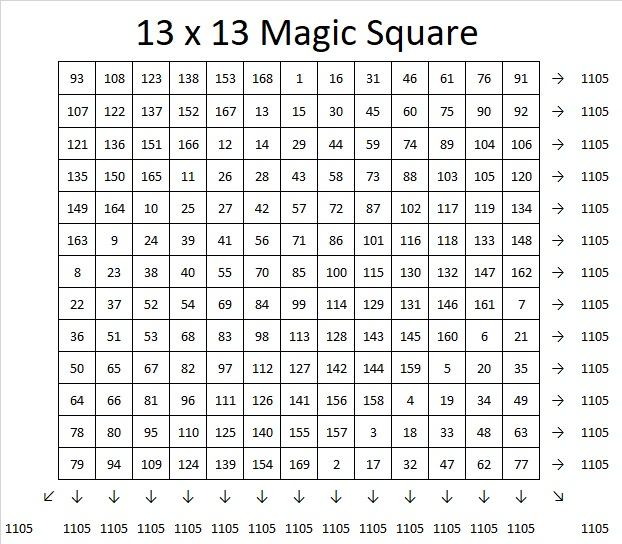If you follow the location of the numbers 1, 2, 3, 4, all the way to 169 in the magic square, you will see the pattern that I used to make that magic square. If you click on 10-factors-1102-1110  and go to the magic squares tab, you can use the same pattern or try another to create an 11 × 11, 13 × 13, or 15 × 15 magic square. The sums on the rows, columns, and diagonals will automatically populate as you write in the numbers so you can verify that you have indeed created a magic square.

1105 tiny squares can be made into a decagon so we say it is a decagonal number: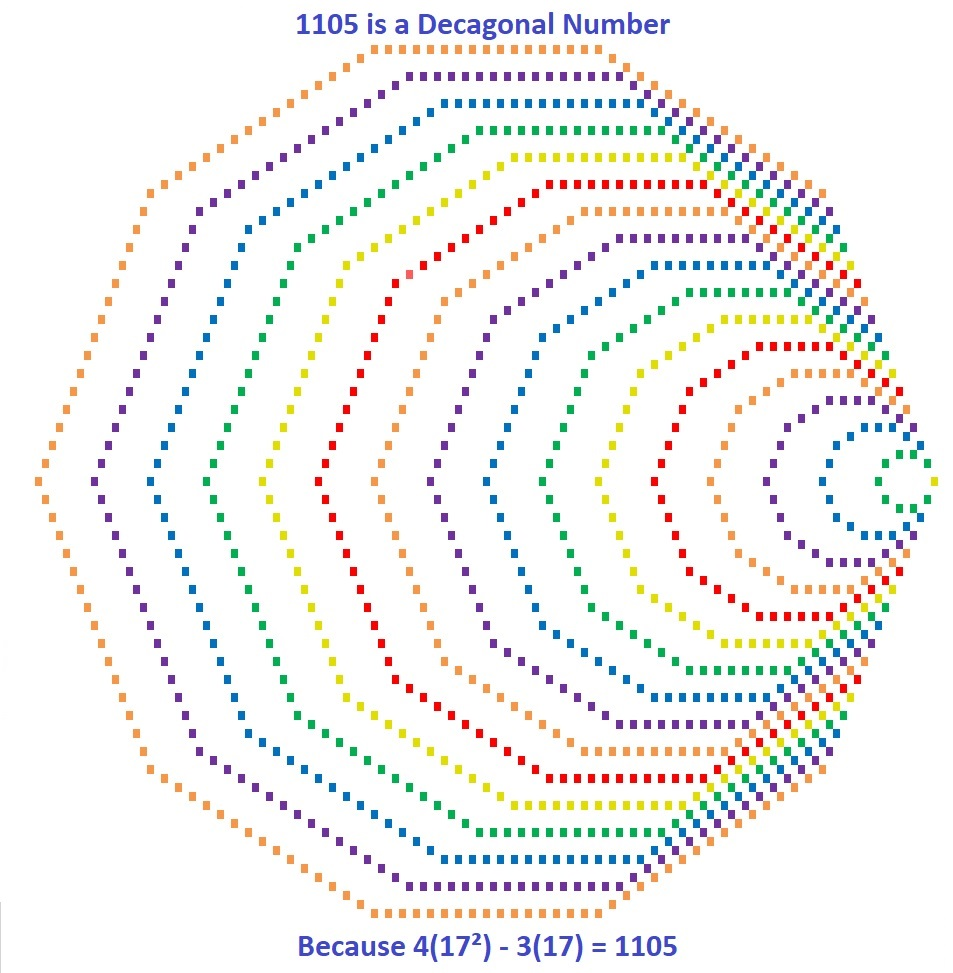Those 1105  tiny squares can also be arranged into a centered square: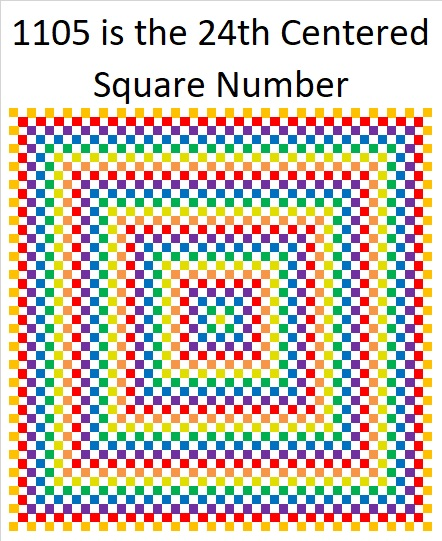Why is 1105 the 24th Centered Square Number? Because it is the sum of consecutive square numbers:
24² + 23² = 1105

But that’s not all! 1105 is the smallest number that is the sum of two squares FOUR different ways:

24² + 23² = 1105
31² + 12² = 1105
32² + 9² = 1105
33² + 4² = 1105

1105 is also the smallest number that is the hypotenuse of THIRTEEN different Pythagorean triples. Yes, THIRTEEN! (Seven was the most any previous number has had.) It is also the smallest number to have FOUR of its Pythagorean triplets be primitives (Those four are in blue type.):

47-1104-1105 calculated from 24² – 23², 2(24)(23), 24² + 23²
105-1100-1105 which is 5 times (21-220-221)
169-1092-1105 which is 13 times (13-84-85)
264-1073-1105 calculated from 2(33)(4), 33² – 4², 33² + 4²
272-1071-1105 which is 17 times (16-63-65)
425-1020-1105 which is (5-12-13) times 85
468-1001-1105 which is 13 times (36-77-85)
520-975-1105 which is (8-15-17) times 65
561-952-1105 which is 17 times (33-56-85)
576-943-1105 calculated from 2(32)(9), 32² – 9², 32² + 9²
663-884-1105 which is (3-4-5) times 221
700-855-1105 which is 5 times (140-171-221)
744-817-1105 calculated from 2(31)(12), 31² – 12², 31² + 12²

Why is it the hypotenuse more often than any previous number? Because of its factors! 1105 = 5 × 13 × 17, so it is the smallest number that is the product of THREE different Pythagorean hypotenuses.

It gets 1 triple for each of its three individual factors: 5, 13, 17, 2 triples for each of the three ways the factors can pair up with each other: 65, 85, 221, and four primitive triples for the one way they can all three be together: 1105. Thus it gets 2º×3 + 2¹×3 + 2²×1 = 3 + 6 + 4 = 13 triples.

Speaking of factors, let’s take a look at 1105’s factoring information:

• 1105 is a composite number.
• Prime factorization: 1105 = 5 × 13 × 17
• The exponents in the prime factorization are 1, 1, and 1. Adding one to each and multiplying we get (1 + 1)(1 + 1)(1 + 1) = 2 × 2 × 2 = 8. Therefore 1105 has exactly 8 factors.
• Factors of 1105: 1, 5, 13, 17, 65, 85, 221, 1105
• Factor pairs: 1105 = 1 × 1105, 5 × 221, 13 × 85, or 17 × 65
• 1105 has no square factors that allow its square root to be simplified. √1105 ≈ 33.24154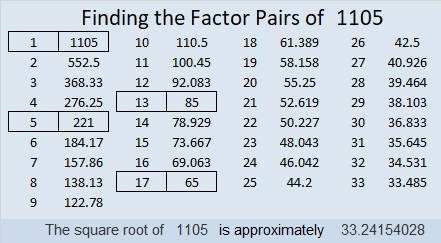1105 is also a palindrome in four different bases, and I also like the way it looks in base 8:
It’s 10001010001 in BASE 2 because 2¹º + 2⁶ + 2⁴ + 2º = 1105,
101101 in BASE 4 because 4⁵ + 4³ + 4² + 4º = 1105,
2121 in BASE 8 because 2(8³) + 1(8²) + 2(8) + 1(1) = 1105,
313 in BASE 19 because 3(19²) + 1(19) + 3(1) = 1105
1M1 in BASE 24 (M is 22 base 10) because 24² + 22(24) + 1 = 1105

Last, but certainly not least, you wouldn’t think 1105 is a prime number, but it is a pseudoprime: the second smallest Carmichael number. Only Carmichael number 561 is smaller than it is.

A Carmichael number is a composite number that behaves like a prime number by giving a false positive to all of certain quick prime number tests:
1105 passes the test p¹¹⁰⁵ Mod 1105 = p for all prime numbers p < 1105. Here is an image of my computer calculator showing 1105 passing the first five tests! Only a prime number should pass all these tests.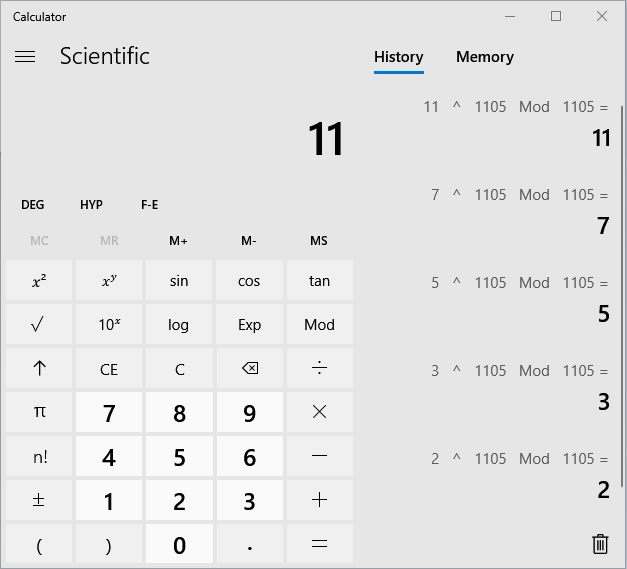1105 is indeed a number with amazing mathemagical properties!

# 561 gives a false positive to these 102 prime number tests

We can use some easy divisibility tests to find two of the factors of 561.

• 5 + 6 + 1 = 12, a multiple of 3 so 561 is divisible by 3.
• 5 – 6 + 1 = 0, which is divisible by 11 so 561 can be evenly divided by 11.

But if we only do those divisibility tests, we will miss something very significant about the number 561:

If you divide 2^561 by 561, the remainder will be 2. When we are more interested in the remainder than the quotient, we can simply type “2, x^y, 561, Mod, 561, =” into the computer’s scientific calculator:This is only a picture of a calculator.

2^561 (mod 561) = 2 means that 561 is VERY LIKELY a prime number, but this is one time when VERY LIKELY does not mean ACTUALLY!

561 has something in common with the number 341. Yes, both of them pass this quick prime number test, and both of them are composite numbers divisible by 11. Both numbers are called pseudo-prime numbers. (341 and 561 are the two smallest composite numbers to give a false positive to this particular test.)

561 is even more remarkable than 341:

• 2^561 (mod 561) = 2, and 2^341 (mod 341) = 2 (Both numbers pass.)
• 3^561 (mod 561) = 3, while 3^341 (mod 341) = 168 (561 passes; 341 fails.)
• 5^561 (mod 561) = 5
• 7^561 (mod 561) = 7
• 11^561 (mod 561) = 11
• 13^561 (mod 561) = 13
• 17^561 (mod 561) = 17
• etc.

There are 102 prime numbers less than 561, and p^561 (mod 561) = p for every single one of them! 561 acts like a prime number in those 102 ways.

In 1910 R. D. Carmichael discovered that 561 is the first COMPOSITE number that passes ALL those modular (remainder) prime number tests, so 561 is the first Carmichael number. Yes, there will be more – in fact, infinitely more.

R. D. Carmichael actually found that 561 passes ALL 559 prime number tests using each whole number between 1 and 561, for example 33^561 (mod 561) = 33. All prime numbers can make a similar claim, but 561 is the smallest composite number with that property.

(Note: I did not use the standard mathematical notation for this property, but what I used is equivalent to it and doesn’t require parenthesis when typing it into the computer’s scientific calculator. Also I think “=” is less intimidating looking for some of my readers than “≡”.)

There are other reasons why the number 561 is an interesting number:

Because 33 x 34/2 = 561, we know that 561 is the 33rd triangular number and is equal to 1 + 2 + 3 + . . . + 31 + 32 + 33, the sum of the first 33 whole numbers.

Because 17 x (2 x 17 – 1) = 561, we know that 561 is the 17th hexagonal number. (All hexagonal numbers are also triangular numbers.)

561 is also the hypotenuse of the Pythagorean triple 264-495-561. What is the greatest common factor of those three numbers? Hint: it is one of the factors of 561 listed below:

——————————————————————–

• 561 is a composite number.
• Prime factorization: 561 = 3 x 11 x 17
• The exponents in the prime factorization are 1, 1, and 1. Adding one to each and multiplying we get (1 + 1)(1 + 1)(1 + 1) = 2 x 2 x 2 = 8. Therefore 561 has exactly 8 factors.
• Factors of 561: 1, 3, 11, 17, 33, 51, 187, 561
• Factor pairs: 561 = 1 x 561, 3 x 187, 11 x 51, or 17 x 33
• 561 has no square factors that allow its square root to be simplified. √561 ≈ 23.6854